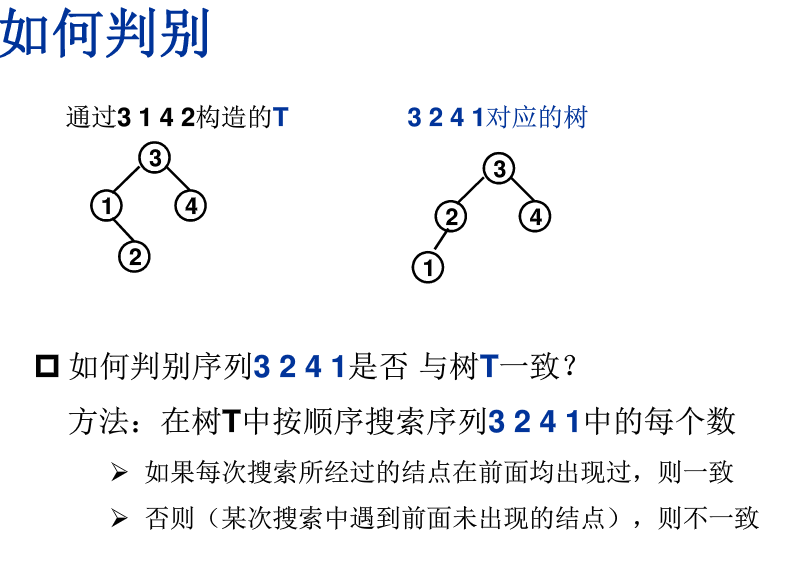# 7-4 是否同一棵二叉搜索树

给定一个插入序列就可以唯一确定一棵二叉搜索树。然而，一棵给定的二叉搜索树却可以由多种不同的插入序列得到。例如分别按照序列{2, 1, 3}和{2, 3, 1}插入初始为空的二叉搜索树，都得到一样的结果。于是对于输入的各种插入序列，你需要判断它们是否能生成一样的二叉搜索树。

### 输入格式:

输入包含若干组测试数据。每组数据的第1行给出两个正整数N (≤10)和L，分别是每个序列插入元素的个数和需要检查的序列个数。第2行给出N个以空格分隔的正整数，作为初始插入序列。最后L行，每行给出N个插入的元素，属于L个需要检查的序列。

简单起见，我们保证每个插入序列都是1到N的一个排列。当读到N为0时，标志输入结束，这组数据不要处理。

### 输出格式:

对每一组需要检查的序列，如果其生成的二叉搜索树跟对应的初始序列生成的一样，输出“Yes”，否则输出“No”。

### 输入样例:

4 2
3 1 4 2
3 4 1 2
3 2 4 1
2 1
2 1
1 2
0


### 输出样例:

Yes
No
No

根据题目意思可得，我们需要判断两个序列是否对应相同的二叉搜索树，求解思路有多种，例如：

1.我们可以分别建立两个二叉搜索树进行判别，分别递归判断其左右子树是否相同；

2.不建树的判别方法；比如对于序列3 1 4 2和3 2 4 1，我们首先比较第一个数字（根结点），若不相同，则肯定不是相同的二叉树；其次把序列1和2中比根结点小的数放在根结点左边，大的放在右边，则序列变为1 2 3 4和2 1 3 4；然后分别比较根结点左边序列和右边序列，看是否相同。

3.建立一棵树，在判别其他序列是否与该树一致。

我们这里主要讲述第3种方法。因此我们的程序框架为：

int main()
{
对每组数据
*读入N和L;
*根据第一行序列建立数T;
*依据树T分别判别后面的L个序列是否能与树T形成同一个二叉搜索树并输出结果;
return 0;
}

那么我们首先需要解决的便是如何建立搜索树T 。我们可以利用二叉搜索树的插入算法，如下：

/**********二叉搜索树插入算法***********/
BinTree Insert(int X,BinTree BST)
{
if( !BST ){  //空树
BST = (BinTree)malloc(sizeof(struct TreeNode));
BST->Data = X;
BST->flag = 0;
BST->Left = BST->Right = NULL;
}else{
if(X > BST->Data)
BST->Right = Insert(X,BST->Right);  //递归插入右子树
else if(X < BST->Data)
BST->Left = Insert(X,BST->Left);    //递归插入左子树
//else X 存在，什么也不做
}
return BST;
}

我们只需要连续插入N次，就可以构成一个搜索树了。

BinTree MakeTree(int N)
{
int x;
BinTree BST = NULL;

for(int i=0; i<N; i++){
scanf("%d",&x);
BST = Insert(x,BST);  //插入法构建二叉搜索树
}

return BST;
}

其次，我们如何判别序列与树T是否一致呢？方法如下：我们如何表征结点在前面出现过呢？只需要给结点的数据结构里加上一个flag标志，flag=1说明前面出现过（访问过），否则则相反。

typedef struct TreeNode *Position;
typedef Position BinTree; /* 二叉树类型 */
struct TreeNode{ /* 树结点定义 */
int Data; /* 结点数据 */
BinTree Left;     /* 指向左子树 */
BinTree Right;    /* 指向右子树 */
int flag;  //用于标记此结点是否被访问过
};

判别函数如下：

int Check(BinTree BST,int X)
{
if( BST->flag ){  /*该结点前面已经访问过（见过）*/
if(X < BST->Data)  return Check(BST->Left,X);
else if(X > BST->Data)  return Check(BST->Right,X);
else return 0;  //序列中有两个相同的数，那么其肯定不与二叉搜索树T相同，返回0
}else{  /*没见过*/
if(X == BST->Data){
BST->flag = 1; //标记为访问过（见过）
return 1;  //一致
}else{
return 0;  //不一致
}
}
}

int Judge(BinTree BST,int N)
{
int x;
int flag = 0;  //flag=0代表目前还一致， 1代表已经不一致

scanf("%d",&x);
if(x != BST->Data)  flag = 1;  //若输入序列的第一个数与根节点不一致，则不是同一棵二叉搜索树
else BST->flag = 1;   //否则，此结点已被访问过，标记记录为1
for(int i=1; i<N; i++){
scanf("%d",&x);
/*如果flag已经为1，则不需要继续做Check()函数，继续读取完此序列的数*/
/*否则，判断Check()函数，若为0，flag = 1*/
if((!flag) && (!Check(BST,x)))  flag = 1;
}
if( flag )  return 0;  //不是同一棵树
else return 1;
}

总的实现程序为：

#include<stdio.h>
#include<stdlib.h>

typedef struct TreeNode *Position;
typedef Position BinTree; /* 二叉树类型 */
struct TreeNode{ /* 树结点定义 */
int Data; /* 结点数据 */
BinTree Left;     /* 指向左子树 */
BinTree Right;    /* 指向右子树 */
int flag;  //用于标记此结点是否被访问过
};

Position MakeTree(int N);
int Judge(BinTree BST,int N);
void ResetTree(BinTree BST);
void FreeTree(BinTree BST);

int main()
{
int N,L;
BinTree T = NULL;

scanf("%d",&N);
while( N ){
scanf("%d",&L);
T = MakeTree(N);
for(int i=0; i<L; i++){
if(Judge(T,N))  printf("Yes\n");
else  printf("No\n");
ResetTree(T);  //清除树中每个结点的flag标记
}
FreeTree(T);   //释放树的结点空间
scanf("%d",&N);
}
return 0;
}

/**********二叉搜索树插入算法***********/
Position Insert(int X,BinTree BST)
{
if( !BST ){  //空树
BST = (BinTree)malloc(sizeof(struct TreeNode));
BST->Data = X;
BST->flag = 0;
BST->Left = BST->Right = NULL;
}else{
if(X > BST->Data)
BST->Right = Insert(X,BST->Right);  //递归插入右子树
else if(X < BST->Data)
BST->Left = Insert(X,BST->Left);    //递归插入左子树
//else X 存在，什么也不做
}
return BST;
}

Position MakeTree(int N)
{
int x;
BinTree BST = NULL;

for(int i=0; i<N; i++){
scanf("%d",&x);
BST = Insert(x,BST);  //插入法构建二叉搜索树
}

return BST;
}

int Check(BinTree BST,int X)
{
if( BST->flag ){  /*该结点前面已经访问过（见过）*/
if(X < BST->Data)  return Check(BST->Left,X);
else if(X > BST->Data)  return Check(BST->Right,X);
else return 0;  //序列中有两个相同的数，那么其肯定不与二叉搜索树T相同，返回0
}else{  /*没见过*/
if(X == BST->Data){
BST->flag = 1; //标记为访问过（见过）
return 1;  //一致
}else{
return 0;  //不一致
}
}
}

int Judge(BinTree BST,int N)
{
int x;
int flag = 0;  //flag=0代表目前还一致， 1代表已经不一致

scanf("%d",&x);
if(x != BST->Data)  flag = 1;  //若输入序列的第一个数与根节点不一致，则不是同一棵二叉搜索树
else BST->flag = 1;   //否则，此结点已被访问过，标记记录为1
for(int i=1; i<N; i++){
scanf("%d",&x);
/*如果flag已经为1，则不需要继续做Check()函数，继续读取完此序列的数*/
/*否则，判断Check()函数，若为0，flag = 1*/
if((!flag) && (!Check(BST,x)))  flag = 1;
}
if( flag )  return 0;  //不是同一棵树
else return 1;
}

void ResetTree(BinTree BST)
{
if( BST ){
if(BST->Left) ResetTree(BST->Left);   //清除左子树标记
if(BST->Right) ResetTree(BST->Right);  //清除右子树标记
BST->flag = 0;          //清除根节点标记
}
}

void FreeTree(BinTree BST)
{
if( BST ){
if(BST->Left) FreeTree(BST->Left);    //释放左子树空间
if(BST->Right) FreeTree(BST->Right);   //释放右子树空间
free(BST);              //释放根节点空间
}
}©️2019 CSDN 皮肤主题: 技术黑板 设计师: CSDN官方博客